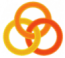General
 Course Home Page ESSLLI 2004Material
Lecture 1
 Slides Practical Session
Lecture 2
 Slides Practical Session
Lecture 3
 Slides Practical Session
Lecture 4
 Slides Practical Session
Lecture 5
 Slides Practical Session

# Solution

```%% The wrapper predicate. Calls pptree/2 with 0 indentation.
pptree(Term) :- pptree(0,Term).

%% pptree/2: The first argument says how much a new line has to be
%% indented, the second argument is the term to be printed.

%% If the term is an atom, print it.
pptree(_,A) :-
atom(A),
write(A).

%% If the term is a complex term, print a newline, print as many
%% blanks as specified by the first argument, print the functor and an
%% opening parenthesis, then call the predicate process_args to print
%% the arguments.
pptree(Ident,C) :-
functor(C,F,A),
A > 0,
nl, tab(Ident),
write(F),write('('),
NewIdent is Ident + 3,
process_args(1,A,C,NewIdent),
write(')').

%% process_args/4: The first two arguments say which argument is
%% currently being processed and how many arguments there are all
%% together. If these numbers are the same, we are processing the last
%% argument. So, just call pptree/1 for this argument.
process_args(A,A,C,Ident) :-
arg(A,C,AthArg),
pptree(Ident,AthArg).

%% If the first argument (X) is smaller than the second argument, then
%% get the Xth argument of the complex term and print it. Then process
%% the remaining args: that is, call process_args with the first
%% argument (X) being increased by 1.
process_args(X,A,C,Ident) :-
X < A,
arg(X,C,XthArg),
pptree(Ident,XthArg),
NewX is X + 1,
process_args(NewX,A,C,Ident).
```